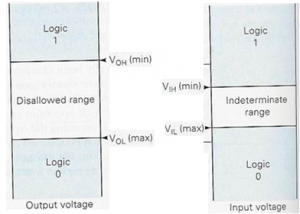Home > digital logic families > noise margin

# Noise Margin

Definition: Ability of the gate to tolerate fluctuations of the voltage levels.The input and output voltage levels defined above point. Stray electric and magnetic fields may induce unwanted voltages, known as noise, on the connecting wires between logic circuits. This may cause the voltage at the input to a logic circuit to drop below VIH or rise above VIL and may produce undesired operation. The circuit's ability to tolerate noise signals is referred to as the noise immunity, a quantitative measure of which is called noise margin.
The noise margins defined above are referred to as dc noise margins. Strictly speaking, the noise is generally thought of as an a.c. signal with amplitude and pulse width. For high speed ICs, a pulse width of a few microseconds is extremely long in comparison to the propagation delay time of the circuit and therefore, treated as d.c. as far as the response of the logic circuit is concerned. As the noise pulse width decreases and approaches the propagation delay time of the circuit, the pulse duration is too short for the circuit to respond. Under this condition, a large pulse amplitude would be required to produce a change in the circuit output. This means that a logic circuit can effectively tolerate a large noise amplitude if the noise is of a very short duration. This is referred to as ac noise margin and is substantially greater than the dc noise margin. It is generally supplied by the manufacturers in the form of a curve between noise margin and noise pulse width.

VNH = HIGH-state noise margin
VNL = LOW-state noise margin
VIL = LOW-state input voltage
VIH = HIGH-state input voltage
VOL = LOW-state output voltage
VOH = HIGh-state output voltage
Where,
VNH = VOH -VIH
VNL = VIL – VOL

See below Figure to calculate noise marginManufacturers specify voltage limits to represent the logical 0 or 1. These limits are not the same at the input and output sides. For example, a particular gate A may output a voltage of 4.8V when it is supposed to output a HIGH but, at its input side, it can take a voltage of 3V as HIGH. In this way, if any noise should corrupt the signal, there is some margin for error.

Noise Margin Quiz:

Noise Margin

1. 22.Which of the following series is a TTL-IC family designed to operate satisfactorily in the temperature range from -550C to +1250C for military applications?